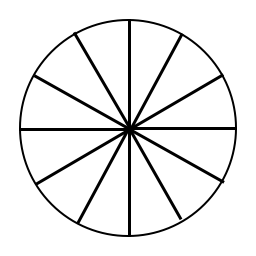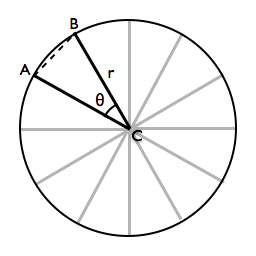## Spokes: A Math Problem

Last night I had a dream that one of my friends had a pair of bike wheels, and asked me a question:

“Which of these two wheels has more spokes?”

In case you’ve forgotten what a bike wheel looks like, it’s something like this:Of course, a real bike wheel has a tire around the outside, and an actual hub at the center. But for our purposes, a bike wheel is a circle with evenly spaced lines (the spokes) radiating out from the origin. This diagram only shows twelve spokes, but a real bike wheel probably has 20 or more—the point being that counting the spokes on the two wheels would have been a tedious way to answer the question.

In the dream, my friend realized it was a tedious task and told me to forget it, but by that point I had already been nerd sniped. Fortunately, a bit of simple geometry makes this question actually quite easy to answer, and I proudly figured it out as I woke up. Here’s the answer:

Measure the distance between the ends of two adjacent spokes1 on each wheel. The wheel whose spokes are closer together has more spokes total.

And here’s why:

1. Since the spokes are evenly spaced, the angles between each adjacent pair of spokes are all the same.

2. The sum of all the angles has to be 2π (or τ, if you prefer).

3. There are as many “angles” as spokes. (You can think of this as going around the inside of the wheel and saying “spoke, angle, spoke, angle…”)

4. Therefore, each angle is τ/n, where n is the number of spokes.

5. Because there are at least two spokes in a real wheel, each angle is at most τ/2, or halfway around the circle. And since we don’t care about direction, each angle is at least 0.

6. The distance between the ends of two spokes grows with the angle. (That is, in the diagram below, making θ bigger will make the distance between A and B bigger.) The reverse is also true, meaning that if A and B get closer together, than θ will get smaller.7. Since θ = τ/n (step 4), a shorter distance (and thus a smaller θ) means a bigger n, i.e. more spokes. QED.

One point I left out is that the two wheels have to be the same size; that is, they have to have the same value for r, the wheel’s radius. If they aren’t the same, though, you can just measure out to the same point on each wheel.

### Behind Step 6

Step 6 is the tricky one that I glossed over. It seems obvious, but I didn’t actually prove it. In this case we can take advantage of the fact that ACB is actually a triangle, and use the generalized form of the Pythagorean Theorem, the Law of Cosines:

In this case, the chord AB is our side c, while AC and BC form sides a and b. And θ is θ, of course.

But wait. We know that the length of a and b are both r, the radius of the circle. So we can rearrange this equation to:

And remember we said that θ is only ever going to be between 0 and τ/2. $\cos(0)$ is 1 and $\cos(\frac{τ}{2})$ is -1, so a smaller θ means a bigger cosine. And since we’re subtracting the cosine, a smaller θ means a smaller distance between A and B.

Which is exactly what Step 6 says.

Postscript: Now that you know how the distance between A and B relates to the wheel’s radius r, you have another way to deal with wheels of different sizes: just divide the distance by r for each wheel.

1. Real bike wheels also usually alternate which side of the wheel the spokes attach to (left and right sides of the bike). But that turns out not to matter for our calculations here; just use two spokes on the same side of the wheel. ↩︎